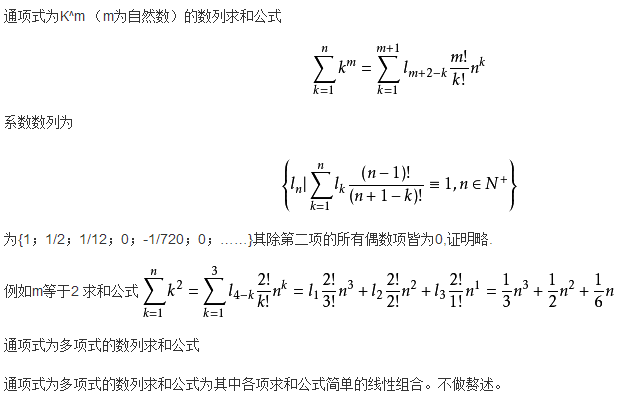# 等差数列求和公式及推导方法## 等差数列公式

1.定义式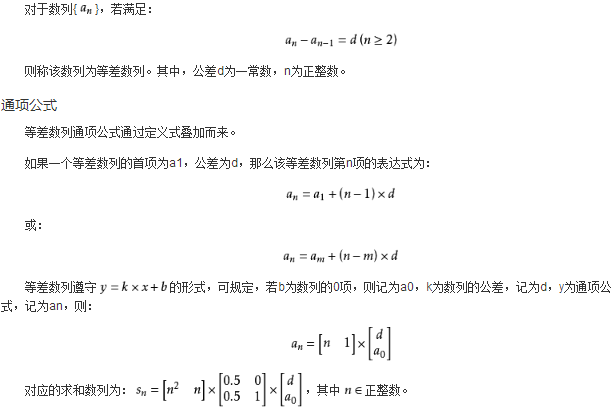2.通项公式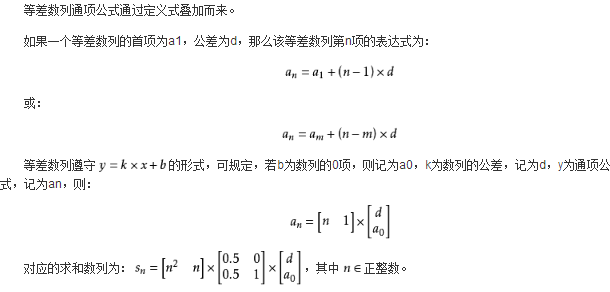3.求和公式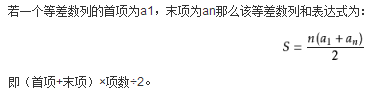4.前n项和公式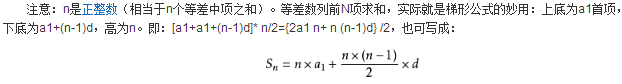## 等差数列推论

（1）从通项公式可以看出，a(n)是n的一次函数(d≠0)或常数函数(d=0)，(n，an)排在一条直线上，由前n项和公式知，S(n)是n的二次函数(d≠0)或一次函数(d=0，a1≠0)，且常数项为0。

（2）从等差数列的定义、通项公式，前n项和公式还可推出：a(1)+a(n)=a(2)+a(n-1)=a(3)+a(n-2)=…=a(k)+a(n-k+1)，(类似：p(1)+p(n)=p(2)+p(n-1)=p(3)+p(n-2)=。。。=p(k)+p(n-k+1))，k∈{1,2,…,n}。

（3）若m，n，p，q∈N*，且m+n=p+q，则有a(m)+a(n)=a(p)+a(q)，S(2n-1)=(2n-1)*a(n)，S(2n+1)=(2n+1)*a(n+1)，S(k)，S(2k)-S(k)，S(3k)-S(2k)，…，S(n)*k-S(n-1)*k…成等差数列，等等。若m+n=2p，则a(m)+a(n)=2*a(p)。

（4）其他推论：

①和=（首项+末项）×项数÷2；

②项数=（末项-首项）÷公差+1；

③首项=2x和÷项数-末项或末项-公差×（项数-1）；

④末项=2x和÷项数-首项；

⑤末项=首项+（项数-1）×公差；

⑥2(前2n项和-前n项和)=前n项和+前3n项和-前2n项和。

## 数列求和方法

1、公式法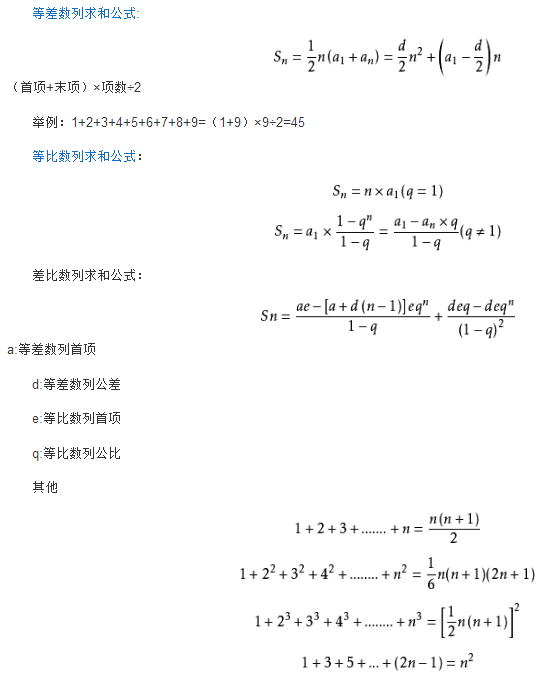2、错位相减法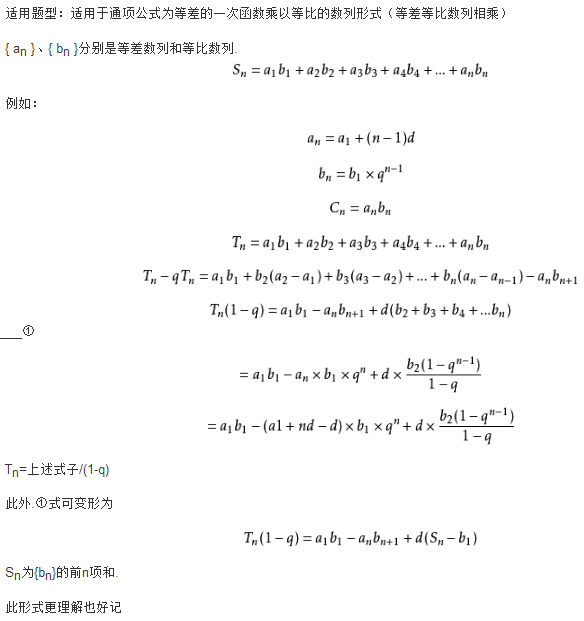3、倒序相加法4、分组法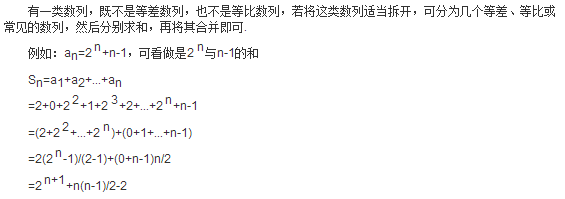5、裂项相消法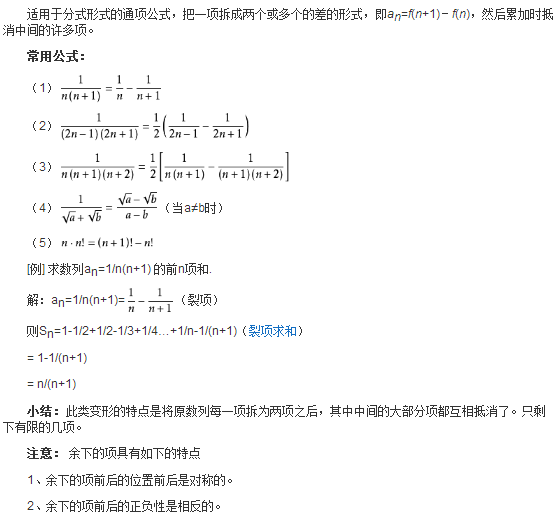6、数学归纳法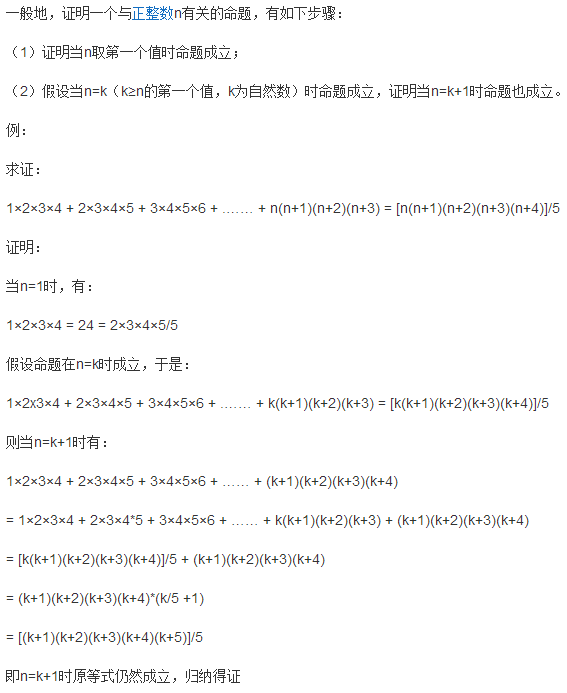7、通项化归法

8、并项求和法

（常采用先试探后求和的方法）

（1－2）+（3－4）+（5－6）+……+[（2n-1）-2n]

an=n(-1)^（n+1）

9、求和公式